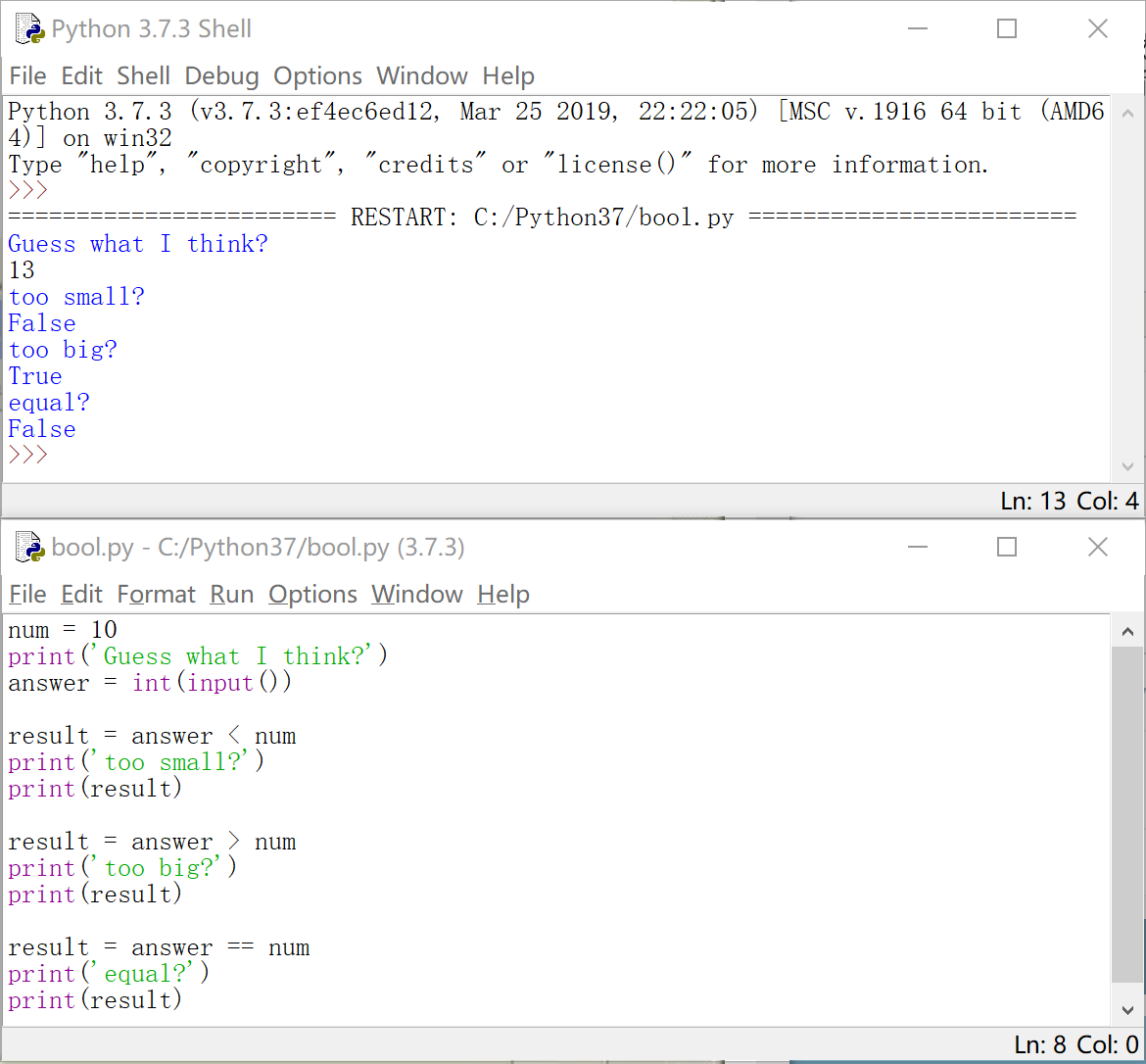## 【Python 第6课】bool

``````a = 1 < 3
print(a)
b = 1
c = 3
print(b > c)``````

“>”“<”在编程语言中被称为比较运算符（或叫 关系运算符），常用的比较运算符包括：

 > 大于 < 小于 >= 大于等于 <= 小于等于 == 等于（比较两个值是否相等。之所以用两个等号，是为了和变量赋值区分开来） != 不等于

 not 逻辑“非” 如果 x 为 True，则 not x 为 False and 逻辑“与” 如果 x 为 True，且 y 为 True，则 x and y 为 True or 逻辑“或” 如果 x、y 中至少有一个为 True，则 x or y 为 True

``````num = 10
print('Guess what I think?')

print('too small?')
print(result)

print('too big?')
print(result)

print('equal?')
print(result)``````#======== 课外的话 ========#